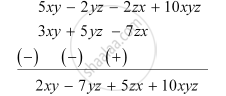Share

# Subtract 3xy + 5yz − 7zx From 5xy − 2yz − 2zx + 10xyz - CBSE Class 8 - Mathematics

ConceptAddition and Subtraction of Algebraic Expressions

#### Question

Subtract 3xy + 5yz − 7zx from 5xy − 2yz − 2zx + 10xyz

#### Solution

The given expressions in separate rows, with like terms one below the other and then the subtraction of these expressions is as follows.Is there an error in this question or solution?

#### APPEARS IN

NCERT Solution for Mathematics Textbook for Class 8 (2018 to Current)
Chapter 9: Algebraic Expressions and Identities
Ex. 9.10 | Q: 4.2 | Page no. 140
Solution Subtract 3xy + 5yz − 7zx From 5xy − 2yz − 2zx + 10xyz Concept: Addition and Subtraction of Algebraic Expressions.
S